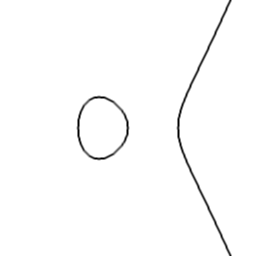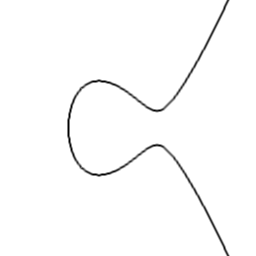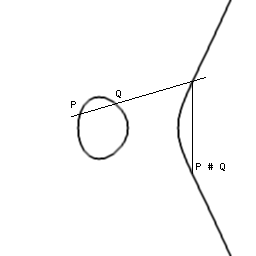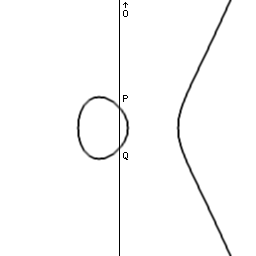# ECDL FAQ - version 0.2

## Index

1. Why is this interesting? Is it all about cracking a code?
2. What is a discrete logarithm?
3. Remind me what a finite group is.
4. What is an elliptic curve and what is the group of points on it?
5. What is a finite field?
6. What is the ECC2K-108 problem?
7. What algorithm can we use to solve ECDL problems like ECC2K-108?
8. What are the iterations in "iterations per second"?
9. What are distinguished points?
10. What are the initial and current expectations?
11. How hard is the ECC2K-108 problem anyway?
12. How can I participate?

## 1. Why is this interesting? Is it all about cracking a code?

By solving ECC2K-108 we are not looking for a secret message but we are looking for a secret key by solving a mathematical problem.

The mathematical problem is called a discrete logarithm. It can be shown that computing discrete logarithms takes exponential time in general, although it can take less in some cases. For elliptic curves no sub-exponential algorithm is known except in rare, specially constructed, examples. Thus it appears that ECDLs are inherently difficult to compute.

This makes them very interesting for mathematicians and programmers: we are constantly searching for better algorithms or implementation methods and expanding the set of special cases. Cryptographers also base the security of codes on the difficulty of computing ECDLs and by solving ECC2K-108 we are demonstrating what elliptic-curve codes can be broken in practice, thereby contributing to improvements in security just as crash-tests improve the safety of cars.

## 2. What is a discrete logarithm?

The discrete logarithm can be defined very generally as follows.

We are given a "thing", called A, and an operation on "things", called #.

For any integer n >= 1, define [n]A to mean A # A # ... # A repeated n times.

The discrete logarithm problem is: given some B of the form [n]A, find n.

We will be concerned with the case where the "things" are in fact elements of some finite group and the operation # is the group operation. There can be several solutions n, but any one will do.

## 3. Remind me what a finite group is.

Unsurprisingly, a finite group is just a group that is finite!

An abelian group (our groups will always be abelian) is a set with an operation # satisfying these properties:

The operation is commutative: A # B = B # A.
There is a neutral element O such that A # O = A, for any A.
Each element A has an inverse I(A) such that A # I(A) = O.

The most familiar example of a group is integers with # being addition. Here O is 0 and I(A) is -A. The discrete logarithm is just division, although we know in advance that the quotient is an integer (there is no remainder).

Another example is positive rational numbers with # being multiplication. Here O is 1 and I(A) is 1/A. The discrete logarithm is an ordinary logarithm, except that we know that the result is an integer.

Examples of finite groups are:
- integers with addition, modulo a positive integer.
- non-zero integers with multiplication, modulo a prime integer.

We will be concerned with the case where the group is the group of points on an elliptic curve.

## 4. What is an elliptic curve and what is the group of points on it?

An elliptic curve is the set of points (x, y) satisfying an equation something like this:

y2 = x3 + a·x + b

The coefficients a, b and the coordinates x, y are taken to be in some field. If it is the field of real numbers, R, then the curve can be drawn on a graph. Two examples are shown.There is a way of defining a group operation on the points. Given two points P and Q on the curve, draw a line through them and find its other intersection with the curve. Then reflect that point through the horizontal axis to find P # Q. When P = Q use the tangent line instead.Now there is one special case when there is no obvious "other intersection" and that is when P reflected through the horizontal axis is equal to Q so that the line is vertical. In this case P is the inverse of Q and so P # Q = O, the neutral group element. But where is O? Well apart from the points on the graph there is one special point called "the point at infinity", and it is O.The operation just described can be proved to satisfy the group properties. It can also be written out using rational functions in the coefficients and coordinates. Note that when the field is not R we may no longer be able to draw nice graphs but the rational functions defining the group operation still make sense and can be used to compute in the group.

We will be concerned with the case when the field is a finite field. Note that the group of points on the curve will necessarily be finite too.

## 5. What is a finite field?

It is a field that is finite, of course!

A field is a set of things with two group operations, addition and multiplication, written + and ·.

The field elements must form an (abelian) group under addition and the non-zero ones must form an (abelian) group under multiplication. Furthermore there is a distributive law like this:

a·(b+c) = a·b + a·c.

Typical examples are rational numbers, real numbers or complex numbers.

An example of a finite field is the set of integers modulo a prime integer. The simplest possible one is the integers modulo 2 i.e., the booleans. Addition and multiplication are XOR and AND respectively. You can check all the field properties if you like!

0 + 0 = 0
0 + 1 = 1
1 + 0 = 1
1 + 1 = 0

0 · 0 = 0
0 · 1 = 0
1 · 0 = 0
1 · 1 = 1

One way to get a large finite field is to take a large prime. Another way is to take a small prime, such as 2, and do a field extension.

For an example of a field extension, start with the boolean field just described. Now we can write polynomials with coefficients in the boolean field. Here is one, with variable t:

t6 + t3 + t2 + t + 1

These polynomials can usually be factored. In this case:

(t2 + t + 1) · (t4 + t3 + 1)
=
t6 + 2·t5 + 2·t4 + t3 + t2 + t + 1
=
t6 + t3 + t2 + t + 1
(modulo 2)

When the polynomial has no factors other than itself or 1, we say that it is a prime polynomial. Here is one:

t109 + t9 + t2 + t + 1

Now we can do arithmetic on polynomials modulo this prime polynomial in roughly the same way as we do arithmetic on integers modulo a prime integer. In this way we get a field with 2109 elements. This is the field used for the ECC2K-108 problem.

## 6. What is the ECC2K-108 problem?

It is an ECDL problem, defined by Certicom, as follows.

Take the field with 2109 elements. The elliptic curve is:

y2 + x·y = x3 + x2 + 1

The points P and Q for which we have to find n with [n]P = Q are:

P = (0x0478C46CC96338CED91565E17257, 0x1E7965E4A3AFB73A48FC9AB790E9)
Q = (0x1FF0CE5EC61893F2119C3077C59E, 0x1F20E9B010AC691C9B87B438241D)

Note that the coefficients of P and Q are really boolean polynomials reduced modulo t109 + t9 + t2 + t + 1 but have been written as hex integers by setting t to 2.

The group of points on this curve has roughly 2109 points (it is always the case that the group size is close to the finite field size). However the ECC2K-108 problem takes place in the sub-group of half the size i.e., roughly 2108 points. The precise number is:

g = 324518553658426701487448656461467

## 7. What algorithm can we use to solve ECDL problems like ECC2K-108?

For computing discrete logarithms in small groups, it is possible to do an exhaustive search of all possible solutions until one works. This method is useless though for a group with well over a hundred thousand billion billion billion elements.

A much smarter way is to use the birthday paradox. It says that if you pick 23 people, then there is more than a 50 % chance that two of them have the same birthday. The reason why only 23 people are needed is that the number of pairs of people grows in proportion to the square of the number of people.

It is the same thing with points on an elliptic curve! If we compute "only" about 30 million billion random points, then chances are good that two will in fact be the same.

We compute points of this form: [s]P # [t]Q with random s and t. If two are the same then:

[s]P # [t]Q = [u]P # [v]Q

so:

[s-u]P = [v-t]Q

so:

[(s-u)/(v-t)]P = Q

So the answer n is (s-u)/(v-t), computed modulo the group size g.

Furthermore the equation of the curve is particularly simple with all coefficients being 0 or 1 (see section 6 above). This is a bad choice for cryptography since it makes the discrete logarithm easier.

It allows us to gather the points into orbits of 2 × 109 = 218 points and use the birthday paradox on whole orbits at a time instead of on individual points. We then need to compute about 2 million billion random orbits and this is roughly ten times faster.

## 8. What are the iterations in "iterations per second"?

To compute points [s]P # [t]Q with random s and t we do not pick new s and t values every time. Computing [s]P # [t]Q from scratch every time would be too slow. Instead we change s and t a bit each time.

For instance if we are at a random point R = [s]P # [t]Q, then we can jump to [2s]P # [2t]Q with a single elliptic operation: R # R.

Instead of just doubling though, we multiply by one of 7 different constants at each step. The choice is made using a pseudo-random hash function. Also, each point is actually a representative of a orbit and the hash function is chosen to be the same for all points in a orbit.

If you run the ecdl2K-108 program and it reports something like 123456 iterations per second, that means that it is jumping from point to point by doing these steps 123456 times every second.

Clearly it would be impossible to send all the points back to base to check for a match between two points. That is why we have distinguished points.

## 9. What are distinguished points?

Distinguished points are those rare random points that happen to have a distinguishing feature (namely that the x coordinate has 23 ones, when written in a certain well-chosen basis). This feature occurs one time in 1.4 billion on average. (The particular feature does not matter much but it must be fixed in advance and it must be the same for all points in a orbit.)

Note that depending on chance it can sometimes take half a billion or 3 billion iterations to find a distinguished point. Don't worry about being "out of luck" though because all points are not quite equal: the value of a point is proportional to the number of iterations it took!

An important thing to notice is that everybody is using the same pseudo-random way of stepping from one random point to another. When somebody eventually finds a random point which is the same as one already visited, then they will follow the exact same steps as the first visitor did, until after a while they find a distinguished point and send it in.

That distinguished point was already found and sent in by the first visitor, so back at base we find the match between distinguished points and compute the answer (s-u)/(v-t) as described in section 7 above.

## 10. What are the initial and current expectations?

We initially expect to need 1.3 million distinguished points. The expectation at any given time is larger than the initial expectation. This is similar to what happens when doing an exhaustive search: at first you expect to examine 50 % of the search space but if you have already checked 20 % without success then you expect to have to go to 60 % instead of 50. We are not doing an exhaustive search but the idea is similar.

Depending on luck, we could find a match after 1 million distinguished points or it might take 2 million but there is no way to tell in advance.

## 11. How hard is the ECC2K-108 problem anyway?

If things work out as expected then the total amount of work will be something like 200000 or 300000 MIPS-years. I believe it is accurate to say:

This is by far the largest public-key crypto crack.
and it is also bigger than all secret-key cracks except possibly for RC5-56 and CS-Cipher done by distributed.net. Indeed:
This is the largest public calculation ever to use a complex distributed algorithm.

where "complex distributed algorithm" is understood to exclude easily parallelized exhaustive searches. In fact it is one of the largest calculations ever, full stop.

In particular, the amount of computation is likely to be about 50% more than needed for secret-key DES cracks. Note that these use exhaustive search and no complex algorithm is required. In the end, one lucky individual finds the DES key. Likewise with RC5. Same thing with finding a new Mersenne prime or a SETI@home block with a message from aliens.

However discrete logarithms (and big factorisations) are different. No single person finds the answer. Ten different projects each doing ten times less work would have little hope of success.

With this ECDL project, the answer can only be found because we are all working together using the exact same algorithm and because we are comparing everybody's distinguished points with everyone else's to look for a match.

Does this project seem awfully difficult? Will we succeed? I think so. But even if for some reason we don't manage, consider this:

The credit belongs to the man in the arena whose face is marred by dust and sweat and blood, who strives valiantly, who errs, and who comes up short again and again, who knows the great enthusiasms, the great devotions, and spends himself in a worthy cause. The man who at best knows the triumph of high achievement and who at worst, if he fails, fails while daring greatly, so that his place will never be with those cold timid souls who never knew victory or defeat.
- Theodore Roosevelt